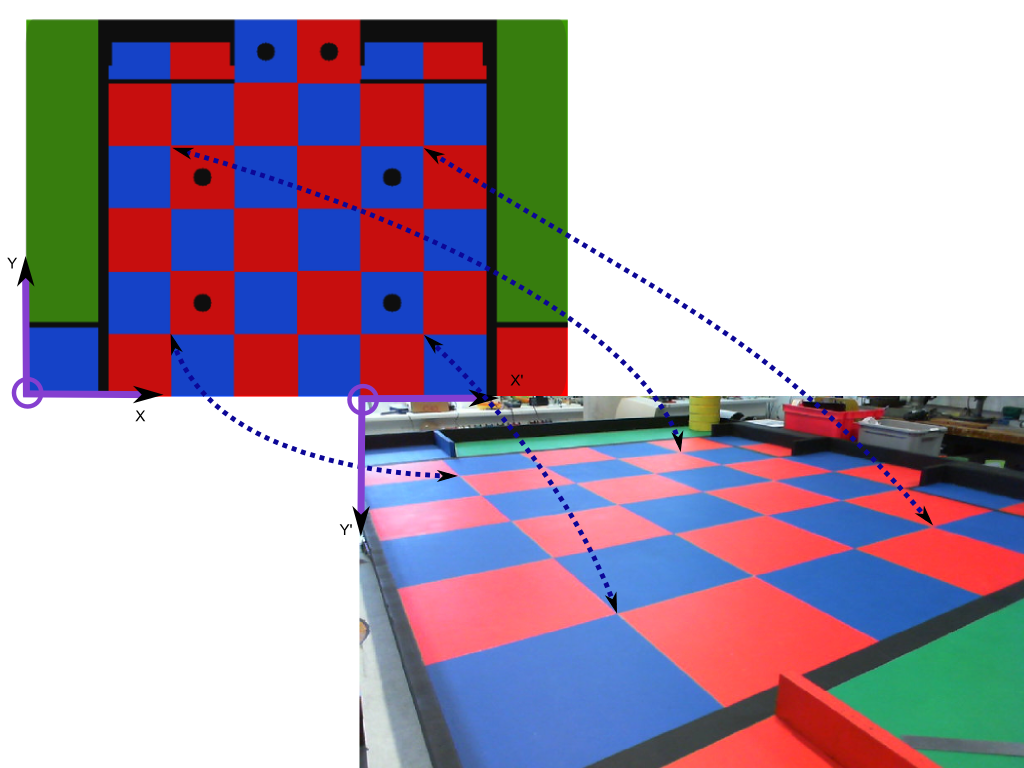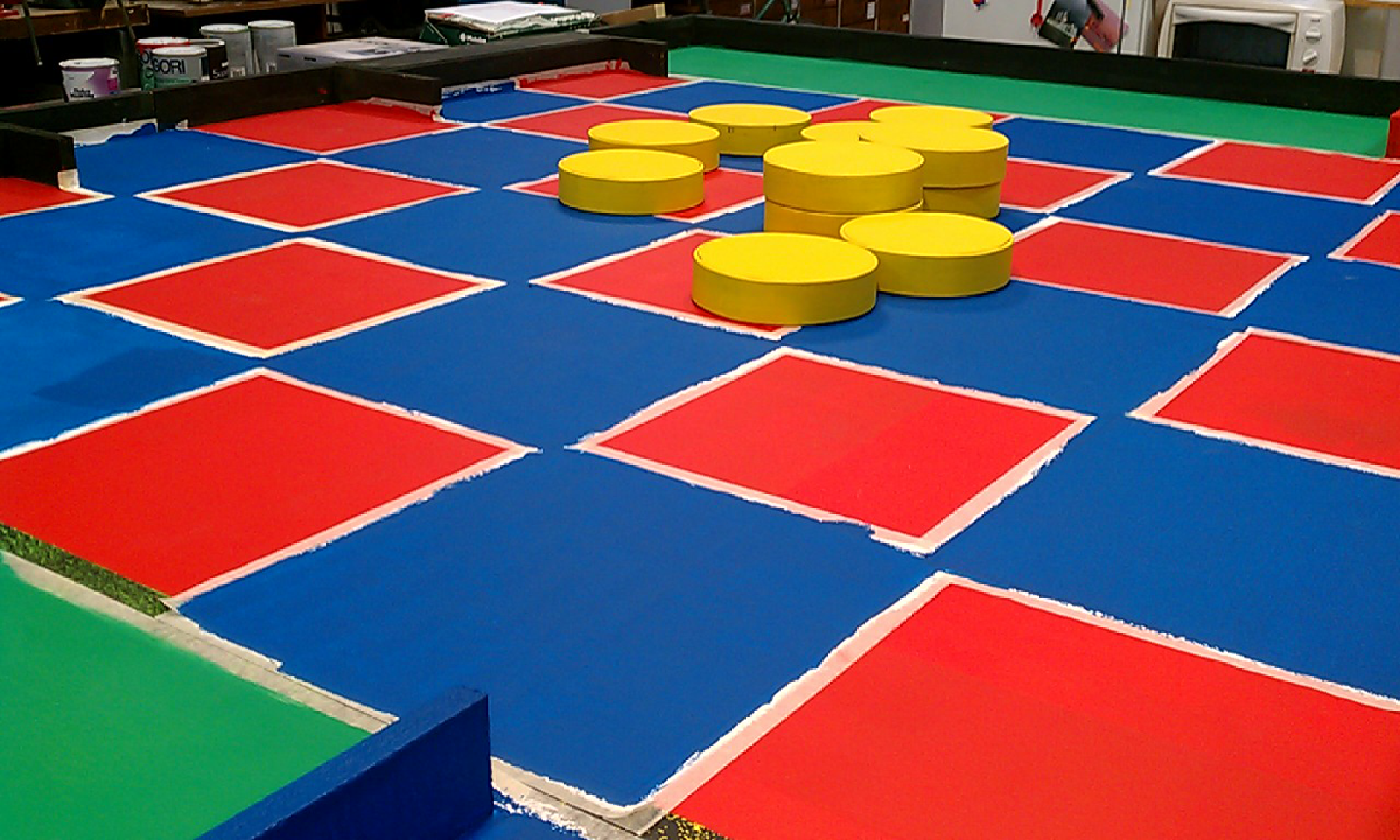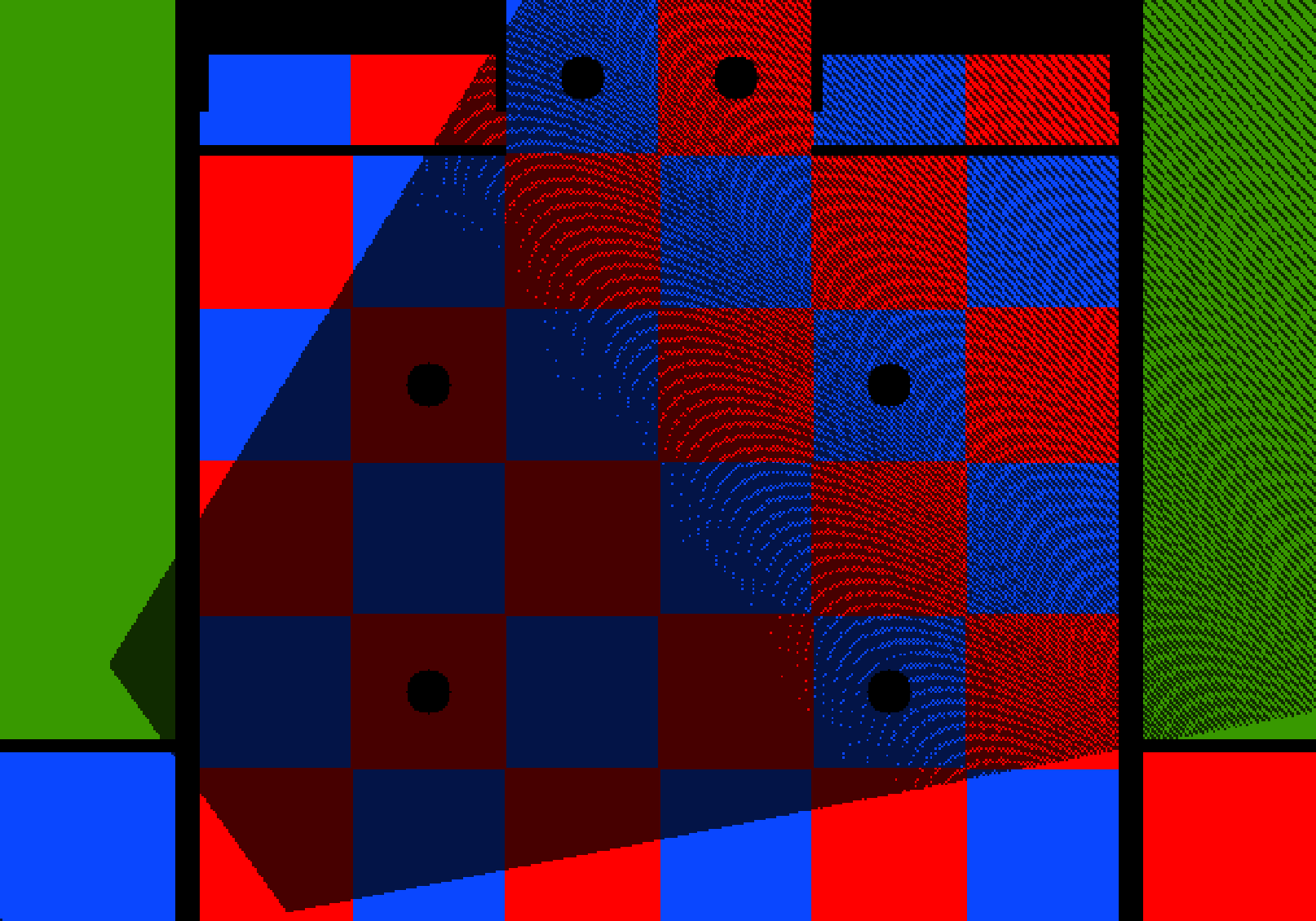## Introduction

With the last step we know where the bottom edges of the pawn are located on the image, we just need to find a way to transform the coordinates of those pixels into game field coordinates.

Given a point $p'$ from the Image plane, we’d like to transform it into $p$ from the Game field plane. We can write:

$p = H \cdot p'$

We observe that straight lines are kept straight, thus H is called the homography matrix which can be computed if at least 4 different matching points are given for both planes. $(p1 \leftrightarrow p1', p2 \leftrightarrow p2', p3 \leftrightarrow p3', p4 \leftrightarrow p4')$It’s worth noticing that both $p$ and $p'$ points are given in homogeneus coordinates.

## Algorithm and code

1. In order to compute H, the homography matrix. We use the ready to use openCV’s function: findHomography.
// Create a column vector with the coordinates of each point (on the field plane)
cv::Mat xField;
xField.create(4, 1, CV_32FC2);
xField.at<Point2f>(0) = ( cv::Point2f(x1, y1) );
xField.at<Point2f>(1) = ( cv::Point2f(x2, y2) );
xField.at<Point2f>(2) = ( cv::Point2f(x3, y3) );
xField.at<Point2f>(3) = ( cv::Point2f(x4, y4) );

// same thing for xImage but with the pixel coordinates instead of the field coordinates, same order as in xField
cv::Mat xImage;
xImage.at<Point2f>(0) = ( cv::Point2f(x1_bis, y1_bis) );
...

// Compute the homography matrix
cv::Mat H = cv::findHomography( xImage, xField );

1. Whenever we want to find the coordinates of a point on the game field, given a pixel $p'(x,y)$ on an image. We only need to transform $p'$ into $p$:
// pImage = p'(x,y)
// pImage is in the projective plane
cv::Mat pImage = (cv::Mat_(3,1) << x, y, 1);
cv::Mat pField = H * pImage;
// pField is in the projective plane (homogeneous coordinates): (X, Y, W). Transform it back to the euclidean plane: (X', Y', 1)
pField       &nbsp;/= pField.at(2);

// p(xField, yField) represent the same point as p'(x, y) but in different planes.
double xField = pField.at(0);
double yField = pField.at(1);


## Optimizations

Because (2) is used really often we can avoid doing matrix products during run time by pre-calculating all possible transformations of the image. All game field points corresponding to every pixel of the image are computed in advance and saved into an bi-dimensional array for efficient access. To correlate $p'$ to $p$ we do:

p = pixelsToMeters.at(p')


## Just for fun

Using the pixelsToMeters array we can print the area the camera sees of the field:## What’s next

You guessed right we now have the full ‘tool-kit’ to precisely link pawns on the image to their positions on the game field plane. How to do it is the subject of the next post.